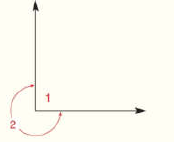Chapter 1.CR, Problem 52CR### Elementary Geometry for College St...

6th Edition
Daniel C. Alexander + 1 other
ISBN: 9781285195698

#### Solutions

Chapter
Section### Elementary Geometry for College St...

6th Edition
Daniel C. Alexander + 1 other
ISBN: 9781285195698
Textbook Problem
1 views

# If m ∠ 1 = 90 ° , find the measure of reflex angle 2 .To determine

To find:

The measure of reflex angle 2 if m1=90°.

Explanation

Given:

The given diagram is,

Given: m1=90°

Calculation:

The measure of reflex angle is between 180° and 360°.

The sum of measure of reflex angle is given by,

m1+m2=360°

Substitute 90° for m1 in the above equation.

### Still sussing out bartleby?

Check out a sample textbook solution.

See a sample solution

#### The Solution to Your Study Problems

Bartleby provides explanations to thousands of textbook problems written by our experts, many with advanced degrees!

Get Started

#### Sketch the graphs of the equations in Exercises 512. x+y=1

Finite Mathematics and Applied Calculus (MindTap Course List)

#### Equations Solve the equation graphically. 140. x+4=x25

Precalculus: Mathematics for Calculus (Standalone Book)

#### In Problems 19-44, factor completely. 41.

Mathematical Applications for the Management, Life, and Social Sciences

#### In Exercises 14, find the slope of the line shown in each figure.

Finite Mathematics for the Managerial, Life, and Social Sciences

#### True or False: converges mean exists.

Study Guide for Stewart's Single Variable Calculus: Early Transcendentals, 8th

#### Solve 4y6=9y+28for y.

Mathematics For Machine Technology